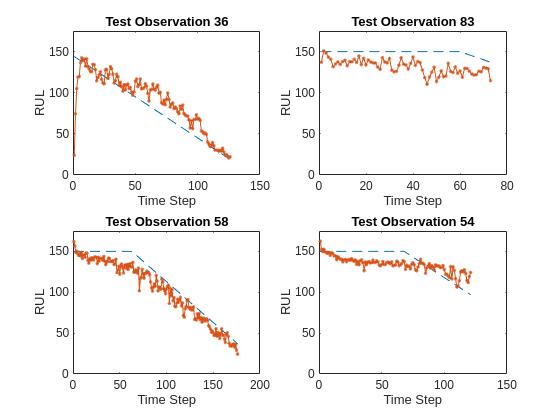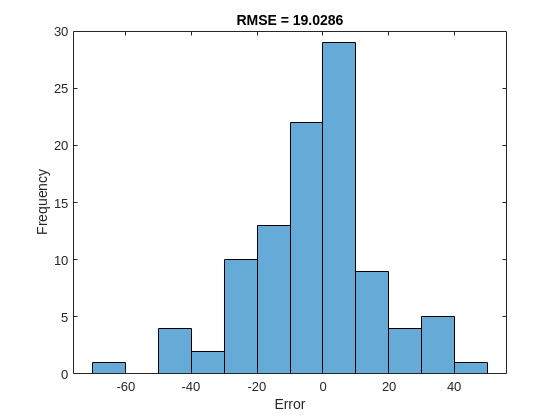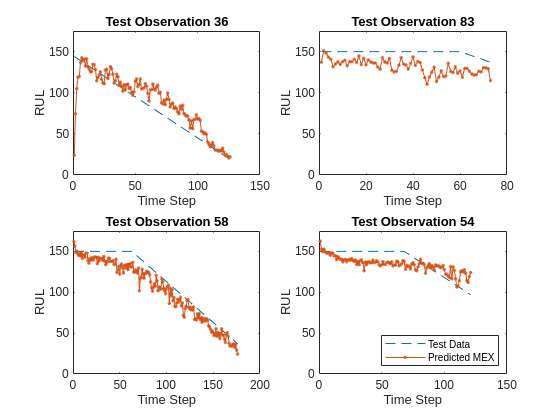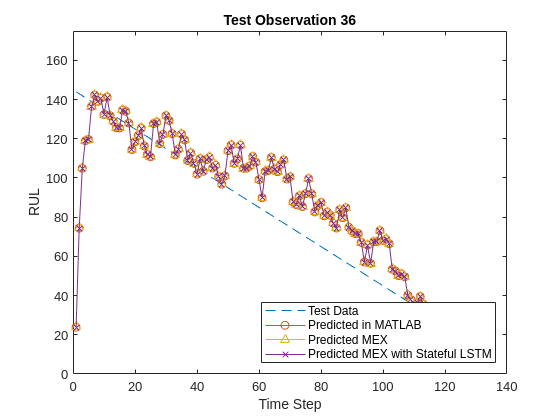# Generate Generic C/C++ Code for Sequence-to-Sequence Regression That Uses Deep Learning

This example demonstrates how to generate plain C/C++ code that does not depend on any third-party deep learning libraries for a long short-term memory (LSTM) network. You generate a MEX function that accepts time series data representing various sensors in an engine. The MEX function then makes predictions for each step of the input timeseries to predict the remaining useful life (RUL) of the engine measured in cycles.

This example uses the Turbofan Engine Degradation Simulation Data Set as described in  and a pretrained LSTM network to predict the remaining useful life of an engine. The network was trained on simulated time series sequence data for 100 engines and corresponding values of the remaining useful life at the end of each sequence. Each sequence in this training data has a different length and corresponds to a full run to failure (RTF) instance. For more information on training the network, see the example Sequence-to-Sequence Regression Using Deep Learning (Deep Learning Toolbox)

### Define Entry-Point Function `rulPredict`

The `rulPredict` entry-point function takes an input sequence and passes it to a trained sequence-to-sequence LSTM network for prediction. The function loads the network object from the `rulNetwork.mat` file into a persistent variable and reuses the persistent object on subsequent prediction calls. The LSTM network makes predictions on the partial sequence one time step at a time. At each time step, the network predicts using the value at this time step, and the network state calculated from the previous time steps only. The network updates its state between each prediction. The `predict` function returns a sequence of these predictions. The last element of the prediction corresponds to the predicted RUL for the partial sequence.

To display an interactive visualization of the network architecture and information about the network layers, use the `analyzeNetwork` (Deep Learning Toolbox) function.

`type rulPredict.m`
```function out = rulPredict(in) %#codegen % Copyright 2020 The MathWorks, Inc. persistent mynet; if isempty(mynet) mynet = coder.loadDeepLearningNetwork('rulNetwork.mat'); end % pass in input to predict method % To prevent the function from adding padding to the data, specify the mini-batch size 1. out = predict(mynet,in,'MiniBatchSize',1); ```

### Run `rulPredict` on Test Data

Load the `TurboFanRULValidate` MAT-file. This MAT-file stores the variable `XValidate` that contains sample timeseries data for sensor readings the you use to test the entry-point function in MATLAB. Make predictions on the test data by calling the `rulPredict` method.

```load TurboFanRULValidate.mat YPred = rulPredict(XValidate);```

Visualize some of the predictions in a plot.

```idx = randperm(numel(YPred),4); figure for i = 1:numel(idx) subplot(2,2,i) plot(YValidate{idx(i)},'--') hold on plot(YPred{idx(i)},'.-') hold off ylim([0 175]) title("Test Observation " + idx(i)) xlabel("Time Step") ylabel("RUL") end legend(["Test Data" "Predicted"],'Location','southeast')```For a given partial sequence, the predicted current RUL is the last element of the predicted sequences. Calculate the root-mean-square error (RMSE) of the predictions, and visualize the prediction error in a histogram.

```YValidateLast = zeros(1, numel(YValidate)); YPredLast = zeros(1, numel(YValidate)); for i = 1:numel(YValidate) YValidateLast(i) = YValidate{i}(end); YPredLast(i) = YPred{i}(end); end figure rmse = sqrt(mean((YPredLast - YValidateLast).^2))```
```rmse = 19.0286 ```
```histogram(YPredLast - YValidateLast) title("RMSE = " + rmse) ylabel("Frequency") xlabel("Error")```### Generate MEX function for `rulPredict`

To generate a MEX function for the `rulPredict` entry-point function, create a code generation configuration object `cfg` for MEX code generation. Create a deep learning configuration object that specifies that no target library is required and attach this deep learning configuration object to `cfg`.

```cfg = coder.config('mex'); cfg.DeepLearningConfig = coder.DeepLearningConfig('TargetLibrary','none');```

By default, the target language is set to C. If you want to generate C++ code, explicitly set the target language to C++.

Use the `coder.typeof` function to create the input type for the entry-point function `rulPredict` that you use with the `-args` option in the `codegen` command.

The data `XValidate` contains 100 observations where each observation is of double data type with a feature dimension value of 17 and a variable sequence length. In order to perform prediction on several such observations in a single function call, you can group the observations together in a cell array and pass the cell array for prediction. The cell array must be a column cell array, and each cell must contain one observation. Each observation must have the same feature dimension, but the sequence lengths might vary as is the case for `XValidate`. Specifying the sequence length as variable-size enables us to perform prediction on an input sequence of any length.

```matrixInput = coder.typeof(0, [17 Inf],[false true]); % input type for a single observation cellInput = coder.typeof({matrixInput}, [100 1]); % input type for multiple observations ```

Run the codegen command. Specify the input type to be `cellInput`.

`codegen -config cfg rulPredict -args {cellInput} -report`
```Code generation successful: To view the report, open('codegen/mex/rulPredict/html/report.mldatx') ```

By default for MEX code generation, the generated code calls into BLAS library for matrix operations and uses OpenMP library (if the compiler supports OpenMP) so that the any parallelizable for loops in the MEX can run on multiple threads leading to better execution performance. While OpenMP is enabled by default for standalone code generation, you will have to provide a custom BLAS callback to indicate to MATLAB Coder ™ that you want to generate BLAS calls for matrix operations following the steps mentioned in Speed Up Matrix Operations in Generated Standalone Code by Using BLAS Calls.

### Run Generated MEX Function on Test Data

Make predictions on the test data by calling the generated MEX function `rulPredict_mex`.

`YPredMex = rulPredict_mex(XValidate);`

You can visualize the same predictions as before in a plot.

```figure for i = 1:numel(idx) subplot(2,2,i) plot(YValidate{idx(i)},'--') hold on plot(YPredMex{idx(i)},'.-') hold off ylim([0 175]) title("Test Observation " + idx(i)) xlabel("Time Step") ylabel("RUL") end legend(["Test Data" "Predicted MEX"],'Location','southeast')```Calculate the root-mean-square error (RMSE) of the predictions, and visualize the prediction error in a histogram.

```YPredLastMex = zeros(1, numel(YValidate)); for i = 1:numel(YValidate) YPredLastMex(i) = YPredMex{i}(end); end figure rmse = sqrt(mean((YPredLastMex - YValidateLast).^2))```
```rmse = 19.0286 ```
```histogram(YPredLastMex - YValidateLast) title("RMSE = " + rmse) ylabel("Frequency") xlabel("Error")```### Generate MEX function with Stateful LSTM

Instead of passing the entire timeseries to predict in one step, you can make predictions one time step at a time by using `predictAndUpdateState` (Deep Learning Toolbox). This is useful when you have the values of the time steps arriving in a stream. The `predictAndUpdateState` function takes in an input, produces an output prediction, and updates the internal state of the network so that future predictions take this initial input into account. Usually, it is faster to make predictions on full sequences when compared to making predictions one time step at a time.

The entry-point function `rulPredictAndUpdate` takes in a single-timestep input and processes the input using the `predictAndUpdateState` function. `predictAndUpdateState` outputs a prediction for the input timestep and updates the network so that subsequent inputs are treated as subsequent timesteps of the same sample. After passing in all timesteps one at a time, the resulting output is the same as if all timesteps were passed in as a single input.

`type rulPredictAndUpdate.m`
```function out = rulPredictAndUpdate(in) %#codegen % Copyright 2020 The MathWorks, Inc. persistent mynet; if isempty(mynet) mynet = coder.loadDeepLearningNetwork('rulNetwork.mat'); end % pass in input to predictAndUpdateState method [mynet, out] = predictAndUpdateState(mynet, in); ```

Run codegen on this new entry-point function. Since we are taking in a single timestep each call, we specify `matrixInput` to have a fixed sequence dimension of 1 instead of a variable sequence length.

```matrixInput = coder.typeof(double(0),[17 1]); codegen -config cfg rulPredictAndUpdate -args {matrixInput} -report```
```Code generation successful: To view the report, open('codegen/mex/rulPredictAndUpdate/html/report.mldatx') ```

Make predictions on the test data by calling the `rulPredictAndUpdate` function in MATLAB an`d the `generated MEX function `rulPredictAndUpdate_mex`.

```YPredStatefulMex = cell(numel(idx), 1); for iSample = 1:numel(idx) sample = XValidate{idx(iSample)}; numTimeStepsTest = size(sample, 2); for iStep = 1:numTimeStepsTest YPredStatefulMex{iSample}(1, iStep) = rulPredictAndUpdate_mex(sample(:, iStep)); end end```

Once again you can visualize the predictions for stateful MEX as before in a plot.

```figure for i = 1:numel(idx) subplot(2,2,i) plot(YValidate{idx(i)},'--') hold on plot(YPredStatefulMex{i},'.-') hold off ylim([0 175]) title("Test Observation " + idx(i)) xlabel("Time Step") ylabel("RUL") end legend(["Test Data" "Predicted MEX Stateful LSTM"],'Location','southeast')```Finally you can also visualize the results for the two different MEX functions along with the MATLAB prediction in a plot for any particular sample.

```figure() sampleIdx = idx(1); plot(YValidate{sampleIdx},'--') hold on plot(YPred{sampleIdx},'o-') plot(YPredMex{sampleIdx},'^-') plot(YPredStatefulMex{1},'x-') hold off ylim([0 175]) title("Test Observation " + idx(i)) xlabel("Time Step") ylabel("RUL") legend(["Test Data" "Predicted in MATLAB" "Predicted MEX" "Predicted MEX with Stateful LSTM"],'Location','southeast')```### References

1. Saxena, Abhinav, Kai Goebel, Don Simon, and Neil Eklund. "Damage propagation modeling for aircraft engine run-to-failure simulation." In Prognostics and Health Management, 2008. PHM 2008. International Conference on, pp. 1-9. IEEE, 2008.